# PRISM CCD testing data reduction package user manual

Contents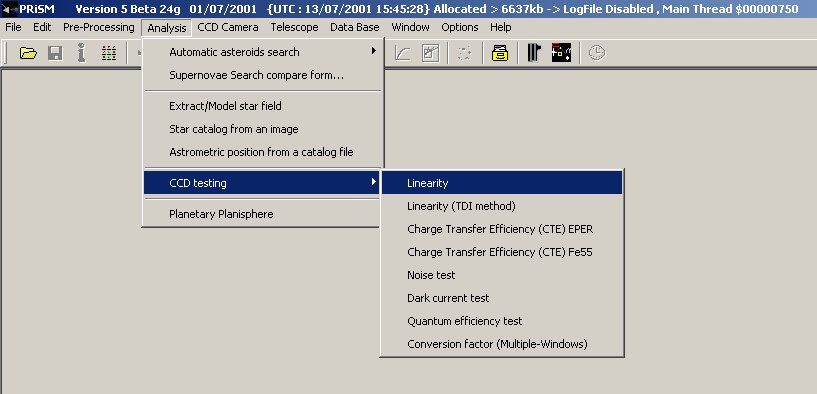Menus of the CCD test data reduction package

#### This user manual is intended to explain how the images have to be acquired and processed with the PRISM's CCD testing package.

The parameters determining  the CCD performances (such as linearity, dark current, conversion factor ...) will not be explained in this page. The user should have the basic knowledge about CCDs. Many books about this subject has been written (see references section).

Those procedures are used at ESO in order to characterize CCD cameras before being installed to the telescope or for CCD preliminary testing.

For a given test, requiring many images, they ALL must have the same amount of pixels (i.e. width/height) and being the same data file type (i.e.: integer or floating point data). Mixtures of image seize and/or data type will result directly in a failure.

### 1. Non-Linearity and Conversion Factor (e-/ADU)

Results

#### The following curves are displayed,  no need to make a table sheet for a plotter like Excel.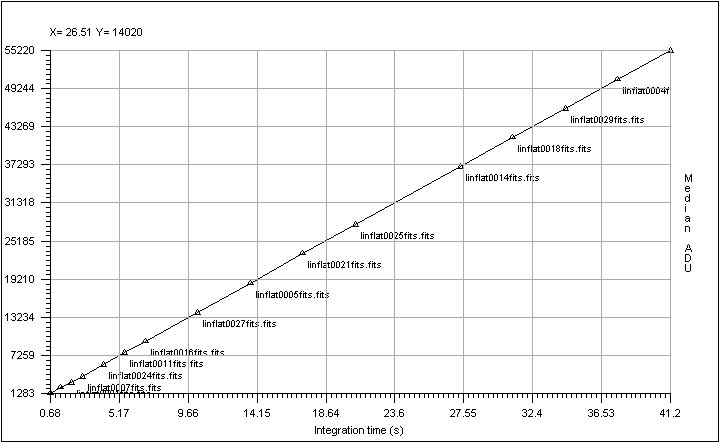Linearity curve according to exposure time.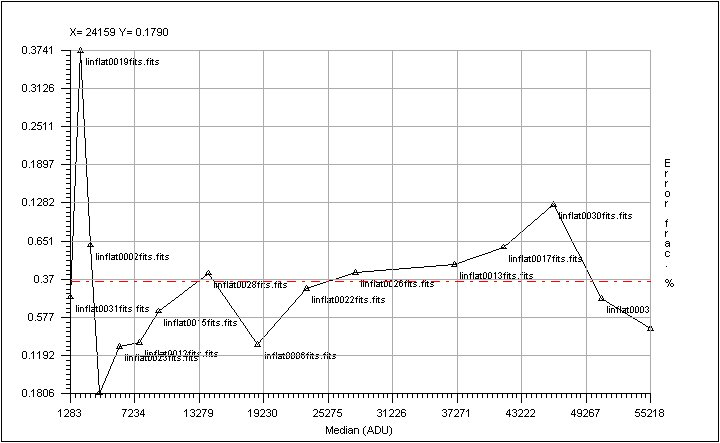#### Residual non linearity curve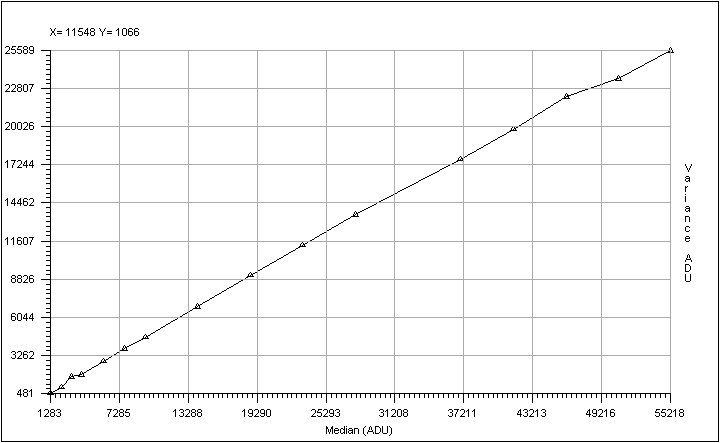#### VERY IMPORTANT : be aware that FITS files are coded sometime as a true 16 bit data (0-65535). PRISM adapts data type according to the input file and to save memory space, but we recommend strongly to open the "Option/FITS options" menu, and to set the "Load 16bits unsigned FITS file to floating point data" as checked.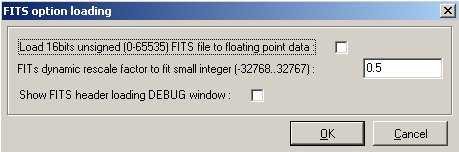## 2. Charge transfer Efficiency (CTE) EPER method

#### For this purpose, acquire a single flat field  image reaching 95% of the ADC dynamic range. The camera shall be able to read the array beyond the photosensitive area. This area beyond the image is called "OVERSCAN", and contains fake pixels having the bias value provided by the electronic readout chain and CCD. This area shall be extended both in X and Y directions. This kind of image has to be provided to PRISM software to compute CTE.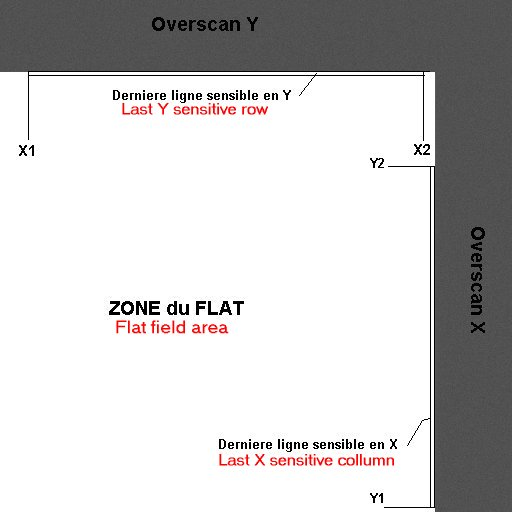#### Y1,Y2 is the area to perform the mean of the last light sensitive row and the X1,X2 is the range to compute the last mean column. The number of transfer across  X and Y is typically the light sensitive image part.

##### Console output: Loading :F:\Images\Frankie\CTE\Cte_0001 Mean -> Last X :58491.6583936574 Last X+1 :469.123061013444 Overscan area :449.922095829024 Horizontal CTE = 0.999999838470179 / 6 nines Mean -> Last Y :65510.6978248089 Last Y+1 :495.093474426808 Overscan area :445.12810646144 Vertical CTE = 0.999999625037444 / 6 nines
Method used :
The method employed here is the EPER (Extended Pixel Edge Response). This method is not really accurate and the IRON 55 tests are much more reliable. Some CTE figures greater than one can be measured with this method !

## 3. Quantum efficiency and PRNU (Photo Response Non uniformity)

#### Once having entered all the calibration files in the software dialog box, the analysis window  X1,X2,Y1,Y2 must be chosen in the way that it must not have any serious defects (black hole, bright pixels).#### The PRISM software provides the quantum efficiency plot as following :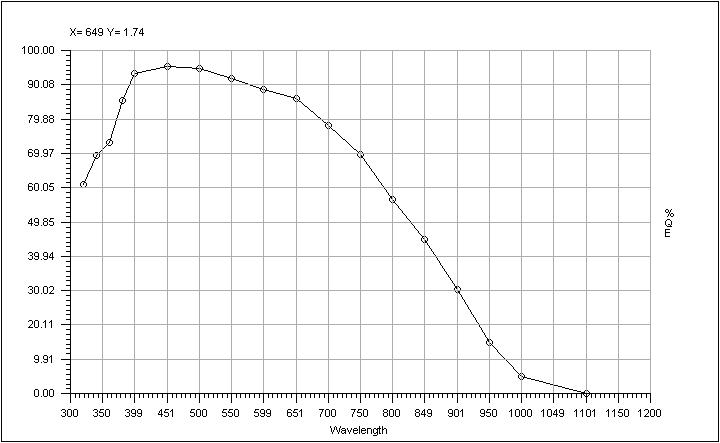#### Also a PRNU curve is provided :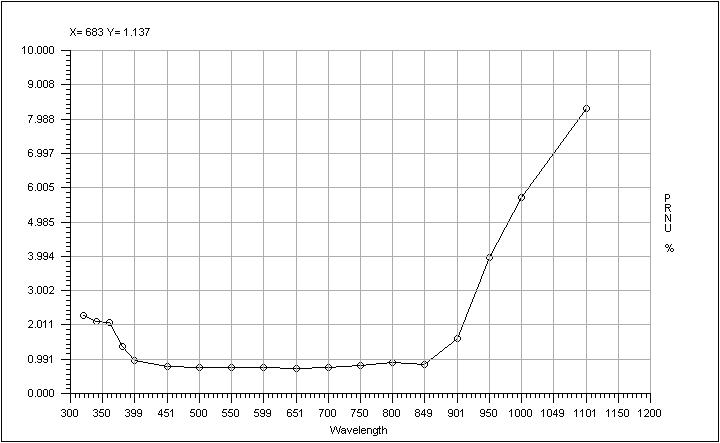#### For instance, this is some images taken at different wavelength of the same area of the CCD (bandwidth=5nm and CCD EEV44 backside illuminated ) :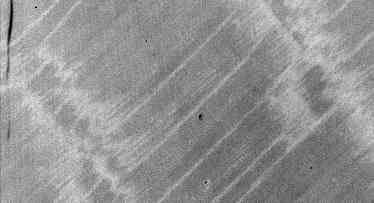at 320 nm (uniformity degraded by the implantation of P+ passivation layer annealed by laser after thinning)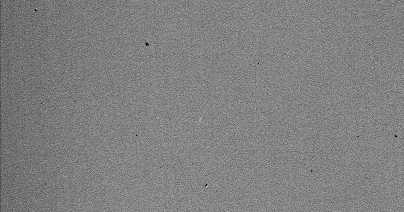at 650 nm (very good uniformity)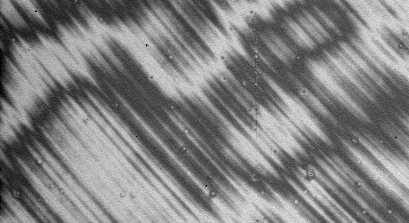at 950 nm (nice fringing !)

## 4. Readout noise

####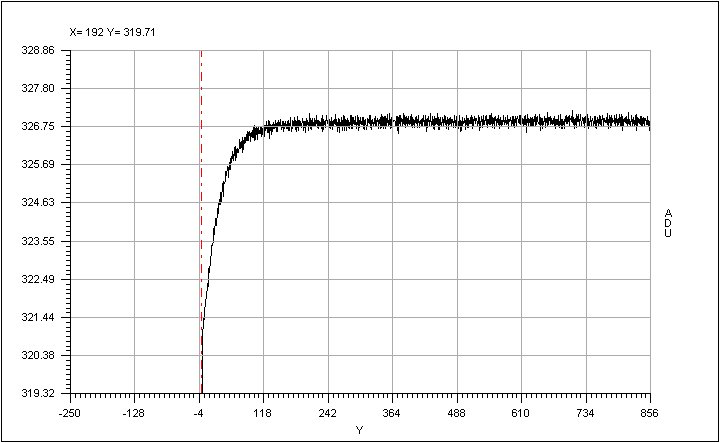The above curve is a stacked column mean over all the columns ,and allows you to display effect that would be drown or hidden by the readout noise .

To trace noise patterns, a Fast Fourier Transform (FFT) of the image is sometime recommended.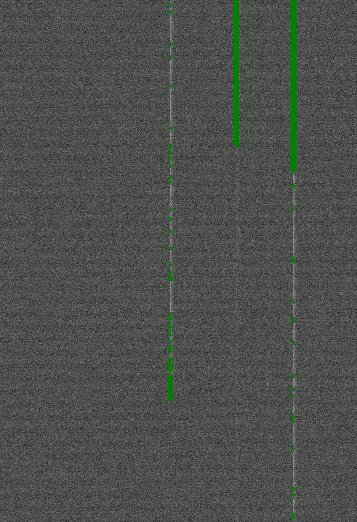#### This image shows cosmetic defects (green cross) over a CCD bias image: these are pixels that are 5 sigma above the median noise + mean.

##### Console output: Pixel amount taken to provide median frame : noise0005 ->1  42.53% noise0003 ->2  57.43% noise0004 ->3  57.65% noise0002 ->4  42.39% Noise : 2.286 ± 0.005463 ADU Pixel amount above 5 sigma : 17100 threshold (ADU) : 6.584

BEWARE : This measurement could be biased if care has not been taken concerning the file format.

Method used :
The RMS value is computed throughout the selected area. The final noise is the median noise from the set of the images. To trace bias defect, a median stack is performed to get rid of cosmic rays, and every pixel which is above or below five (5) sigmas is referenced as a bad pixel and mapped.

See also this

## 5. Dark current

####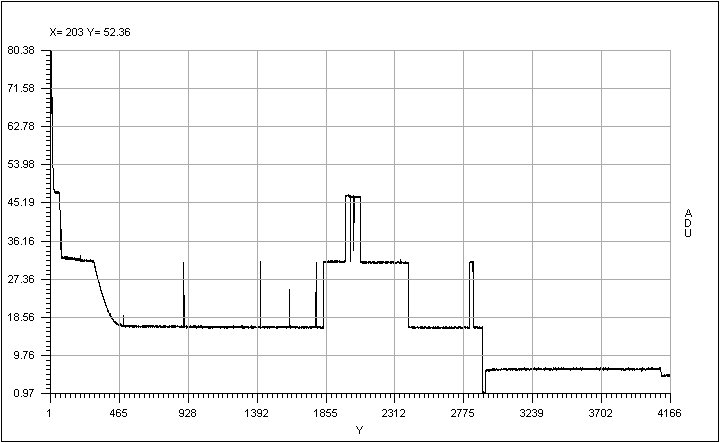This is the mean of all the columns sent to a single resulting column. The steps in the curves shows the defect induced by defect columns.. The same is displayed for the rows.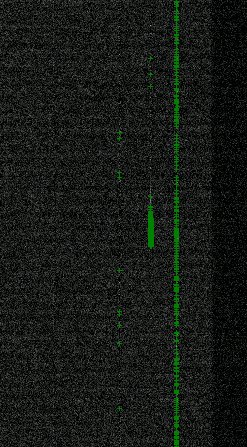#### Here hots pixels map is provided. All pixels above 5 sigma noise+mean are shown and could be regarded as defects.

##### Exposure time (s) =  1800 Dark current : 1.333 ± 0.4714 ADU Dark current : 5.333 ± 1.886 e-/hour/pixel Cosmic event rate : 1.195 ± 0.04297 events/min/cm² Pixel amount above 5 sigmas : 10659 threshold (ADU) : 10.51 Dark current using median frame after median filtering : 5.128 e-/hour/pixel

Even, the software is able to provide the cosmic ray hit rate automatically.

Method used :
The software compute a median stack out of the N provided images and subtract the bias file to it. The dark current is the remaing amount of adus above the bias image (positive data). the defects are subtracted automatically as the difference between individual frames and the median stacked frame.

## 6. Linearity using TDI method

#### This method provides the same results as the classical method, the differences are the following :

• Only one image is necessary (whereas the other method requires many images and takes long time to be carried out).
• Absolutely insensitive to shutter errors.
• The output curve providing the residual non-linearity versus the measured signal is continuous  (whereas the other method provide discrete points)
• The measurement accuracy is much better than the one obtained by the classical different exposure method.
• Not limited by the PRNU of the CCD.

#### This method has, nevertheless, constraints, where the main drawbacks are :

• The ability to open the shutter while the CCD readout process has already began, moreover the shutter must be placed at the entrance of the integrating sphere, but in any case to share the same focal plane as the CCD. This, sometimes, can not be achieved with CCD controllers that does not allow to open the shutter during the readout process. Nevertheless, manual opening can be used if the CCD is not read out to fast.

The method consists in illuminating the CCD by flat field illumination as uniform as possible. The intensity of this flat field light (called here Flux=Fl) must be such as, if T is the CCD readout time and if the CCD is exposed during a T exposure time yielding to a light intensity of Fl, the resulting image must be like a flat field of intensity close to 95% the full ADC dynamic.
In clearer words, For instance, if the dynamic is equal to 16bits, the image must shows up a uniform spatially Flat Field of about 62000 ADUs using the T exposure time and a flux equal to Fl.
Let's assume that the flux has been set (neutral filter, slit settings) so as to get 62000 ADUs in 20sec exposure time. It means that the CCD MUST be clocked in the way that it takes about 20 sec to read it out to achieve TDI linearity method.

The image used for the measurement must be acquired using this way : CCD is started to be readout, shutter being closed, and once the readout of the first 100 first row has been achieved, the shutter is opened (the shutter open delay must be neglected compared to the row readout time). The CCD must be let reading out, being continuously illuminated with a flux equal to Fl. It is advised to hide also the first 100 rows using a mask (light shielded), because the readout circuitry might be disturbed (depends on the CCD) during readout by the continuous light flux to it, and might bias the results.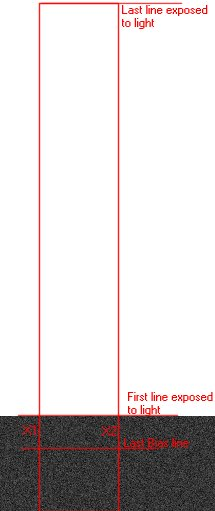Resulting image, display cuts are set to +/-1% of the Bias level. Same image as the right one, with display cuts having the full ADC dynamic range from 0 to 65535, the ramp must be uniform and smooth.

#### So as to reduce this data, the dialog box has to be filled up as following :

• X1 and X2 horizontal defines the vertical stripe.
• The last and the first row exposed to light (sometimes it's better to take the 5th row exposed to light)
• The last row which defines the CCD Bias frame level.
• Minimum flux value is meant to threshold the lower range of the intensity dynamic, to be taken into account for computations and plots.
• Filter : sets how far the output plot curve will be filtered.
• Flat field image is mandatory to correct the data from pixel to pixel non uniformity, it must be an image taken with the same wavelength and bandwidth as the TDI image and subtracted from by its bias image.
• Folder : location of the flat field folder (TBC)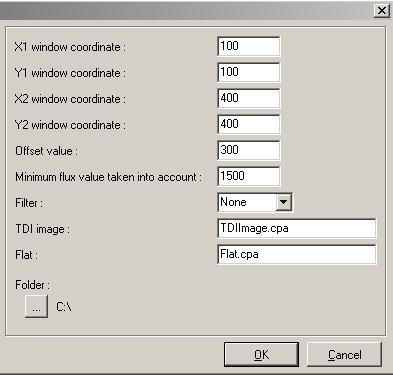Results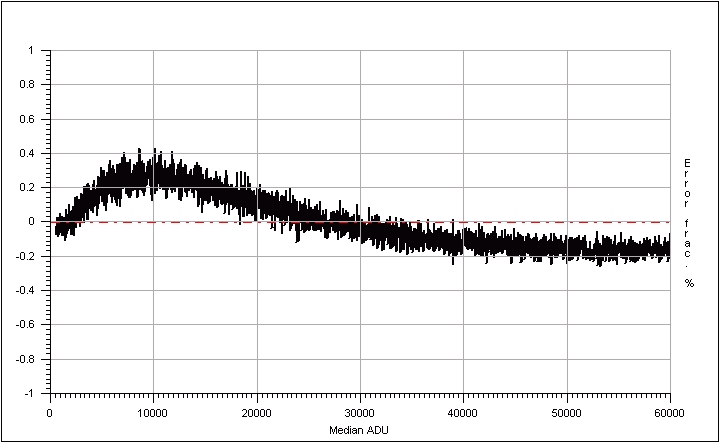Residual non linearity curve, this CCD is  linear with +0.43/-0.3 peak-peak and 0.16% rms deviation from the perfect slope.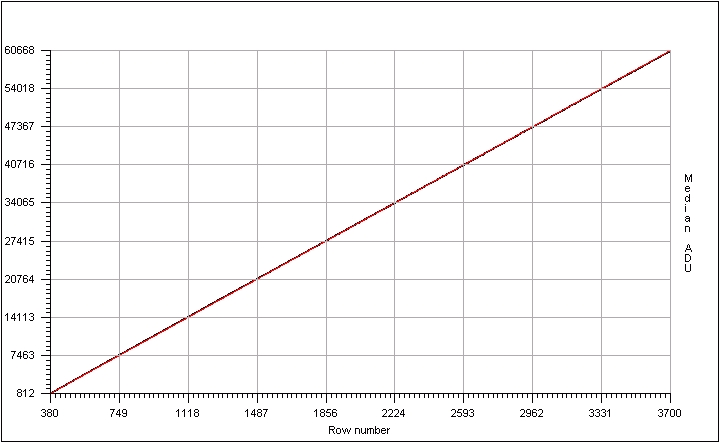This curve is a vertical cross section from the selected area, more exactly the median across this area.

##### Console output: Loading :G:\CCDtest\UvesRed\NewLin\eevLeftPort.cpa Regression slope :17.978;  regression Offset  :-5956.5 Regression slope :18.028 Regression optimum Offset : -6037 Non linearity (Peak to peak) : 0.43% / -0.2912% Non linearity (Mean dev.) : -1.875E-13%  / rms dev 0.1569%

Method used :
The TDI image is median collapsed towards a single column : a 1D slope is achieved. The flats field image is used to correct the slope from the fact that all the pixels of the CCD does not have the same sensitivity. A best linear fit is found from the slope and non-linearity plot computed. Beware that an infinity of slope can be found out of a cloud of points, depending on the criteria : less mean square, weighted points, etc etc...

References :
None : this is a new method developed at ESO.

## 7. Conversion factor using two bias and two flats method

This method is very useful for computing the conversion factor during system development because it is fast and the accuracy is pretty good.
It just needs two biases and two flat field images. It performs conversion factor measurement using NxM sub windows to avoid any problems due to local defects. PRISM asks for the amount of windows that is needed across the X and Y axis. Note that subwindows less than 50x50 pixels can lead to false results, so for a 1x2K CCD for instance, set 10 windows for the X direction and 20 for the Y direction. To remove any prescan/overscan area set the X1,Y1,X2,Y2 windows so as to avoid them. then select the files.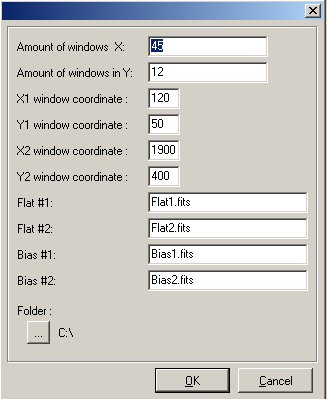##### Console output: Loading :Flat1.fits Loading :Flat2.fits Loading :Bias1.fits Loading :Bias2.fits For all the windows (540) the results are the following : window 1=4.49799e-/ADU X1=120 Y1=50 X2=158 Y2=78 window 2=4.11412e-/ADU X1=120 Y1=79 X2=158 Y2=107 window 3=4.31269e-/ADU X1=120 Y1=108 X2=158 Y2=136 window 4=4.14792e-/ADU X1=120 Y1=137 X2=158 Y2=165 window 5=4.0549e-/ADU X1=120 Y1=166 X2=158 Y2=194 .......... window 536=4.45689e-/ADU X1=1836 Y1=253 X2=1874 Y2=281 window 537=4.55087e-/ADU X1=1836 Y1=282 X2=1874 Y2=310 window 538=4.23658e-/ADU X1=1836 Y1=311 X2=1874 Y2=339 window 539=4.01395e-/ADU X1=1836 Y1=340 X2=1874 Y2=368 window 540=4.50764e-/ADU X1=1836 Y1=369 X2=1874 Y2=397 Conversion Factor=4.3775e-/ADU ± 0.012608 for 3457.054ADU RMS noise =7.0774e- ± 0.092687

Method used :
Note that the algorithm supports flats fields that have different levels of illuminations, tests have been carried out with flats having means with factor of 50 between the two images and the feature passed it pretty well ! This method subtracts the biases from the flat field images, divides the two previous flat images and computes the RMS value (named N here) and the mean Signal for each window. Then computes the D=2*S/N^2 figure, from D, the bias noise is removed and yields to the conversion factor. The corrections due to the different flat field levels are performed by the software, but not mentioned in this explanation (to remain clear).

## 8. Charge transfer efficiency using Fe55 source

This is a very powerful experiment used to derive the vertical and horizontal CCD charge transfer efficiency (CTE) and also can provide a extremely accurate measurement of the conversion factor. An Iron (Fe55) radioactive source is installed 100 mm from the CCD, in the vacuum. This source produces X-rays (5.9Kev photons) that reaches the CCD surface, creating inside the CCD bulk 1620e- in a 0.5/1um sphere. If those 1620e- are produced within a single pixel, they should be detected at the output of the CCD readout node as 1620e- times the conversion factor. Because of "failures" during the charge transfer, some electrons are lost and remains in the next pixel, and this is not . This can happen either during the vertical transfer or the horizontal transfer (serial register). Since the amount of electrons produced by the Fe55 are well defined, it is possible to compute the horizontal and vertical CTE.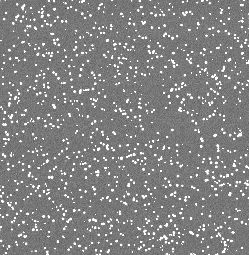A 30 sec exposure image, CCD in front of a Fe55 source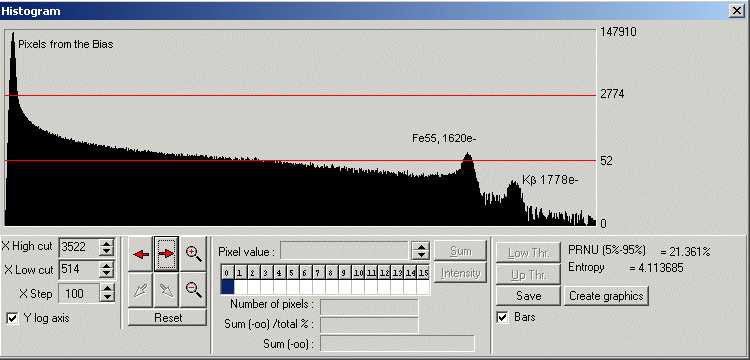Histogram plot of the whole CCD, pixels from the bias level and the two peeks of Fe55 (Ka1620e- Kb1778e-) are visible.

In the dialog form, enter the window to be processed (X1,Y1,X2,Y2). Then the readout direction (CCD output port direction left/right). The conversion factor must be known within 5% accuracy. The bias offset level as also to be provided (accurate, from overscan areas or master bias frame). Select more than one image is recommended for better accuracy, as so as to enter more than 2 lines for each packet. Those packet are used to bin the histogram of a line/column N times.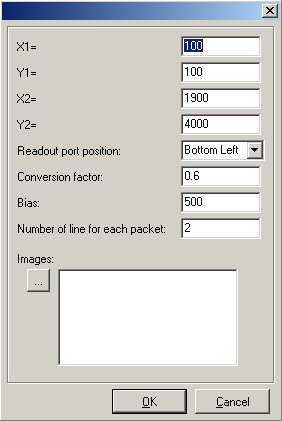Results

##### Console output: Load image: iron-1.cpa Load image: iron-3.cpa Load image: iron-2.cpa Load image: iron-4.cpa CTEV=1.000000101 CTEH=0.999997222 Conversion factor=0.680809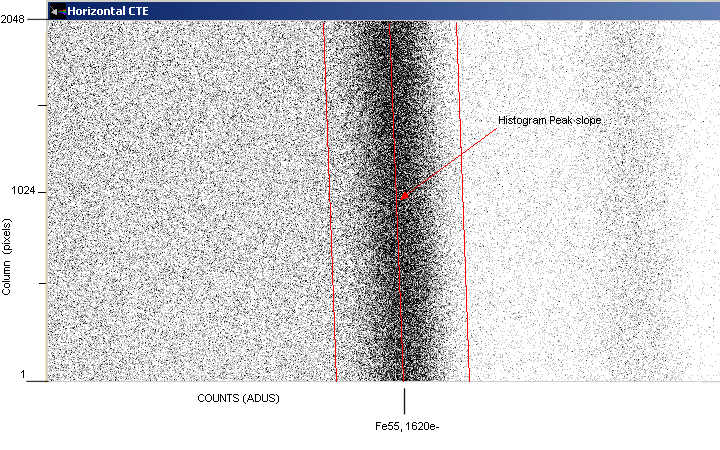Horizontal CTE histogram as an image the fact that the slope is left tilted showed that CTE is lower than 1.0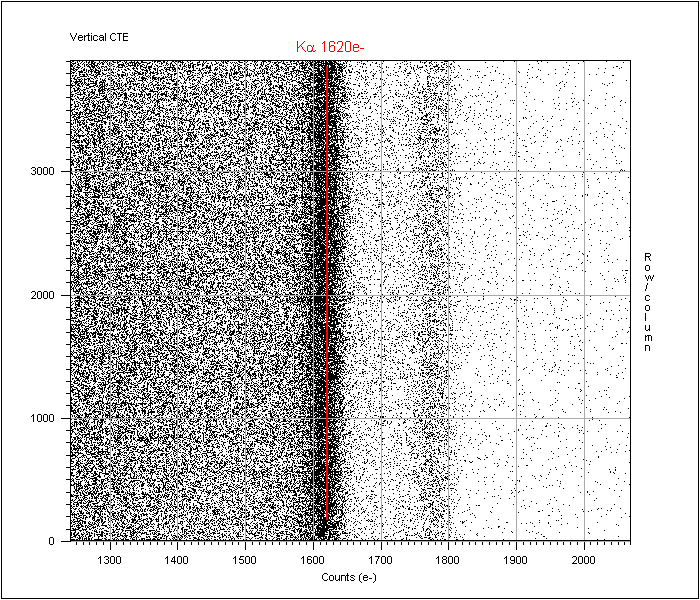Vertical CTE histogram as a printable plot (very good V.CTE)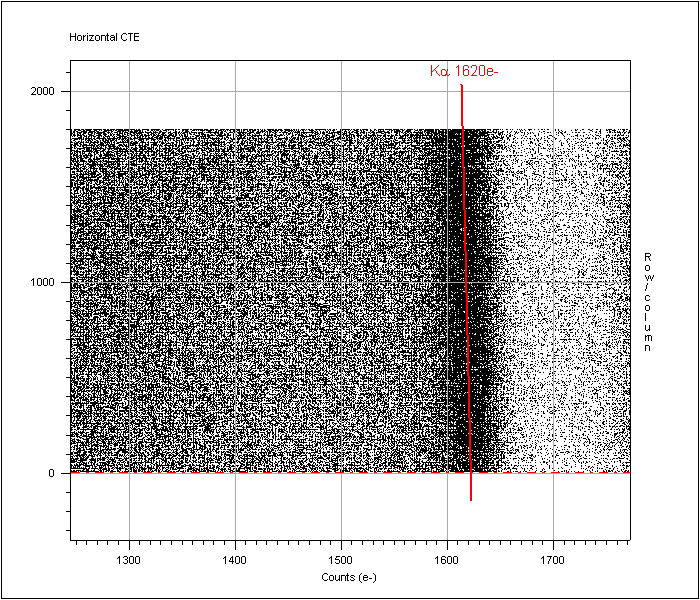Horizontal CTE histogram as a printable plot (H.CTE =0.999997222 )

Method used :
The software performs vertical histogram gathering N columns so as to have a better Signal to Noise measurement, it also uses more than one image to improve the measurement Then displays an image where the X axis is the counts in ADUs, Y the column number and Z the number of pixels having the given X counts.
The softwarefinds the histogram peaks for every column and fits the best slope. As you can see on the image above, the slope that joins all the peak is slightly tilted to the left, showing that the 1620e- created at the end of the serial register (column 2048) are indeed less than 1620e-, thus showing a CTE not equal to 1. The software, according to the histogram peak slope can compute the HCTE.

The same method applies for the horizontal CTE, just swap the word vertical with horizontal and the word column with rows.

References section

1. Electronic imaging in Astronomy, by Ian S.McLean
2. Scientific Charge Coupled Devices, by J.Janesick
3. Optical CCD Detectors - CCD Tests and Characterization at ESO Garching
4. Astronomical CCD Observing and Reduction Techniques
Ed. Steve B. Howell
5. In situ CCD testing

This file was updated by C.Cavadore, 17/02/2006